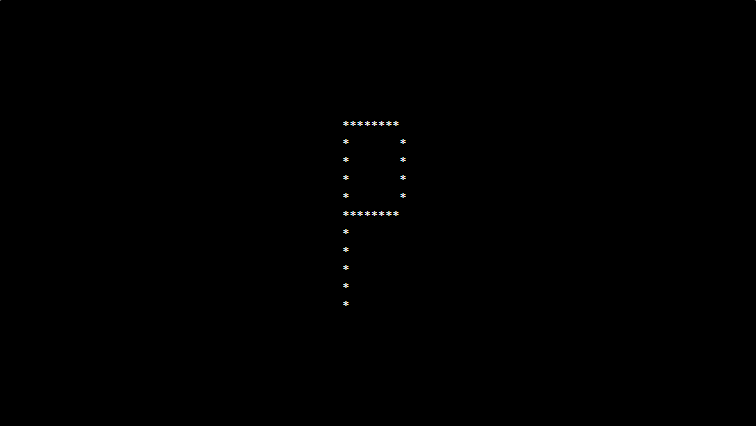# PHP program to print alphabet pattern P

In this article write a PHP program to print alphabet pattern P. This Program first takes the numbers of rows and then prints pattern using nested for loops.

## PHP program to print alphabet pattern P

```<?php
echo "<pre>";
for (\$row = 0; \$row < 11; \$row++) {
for (\$col = 0; \$col <= 11; \$col++) {
if (\$col == 1 OR ((\$row == 0 or \$row == 5) AND \$col > 0 AND \$col < 9) OR ((\$col == 9 OR \$col == 1) AND (\$row > 0 AND \$row < 5))) {
echo "*";
} else {
echo "&nbsp;";
}
}
echo "<br/>";
}
echo "</pre>";
?>
```

### Output: